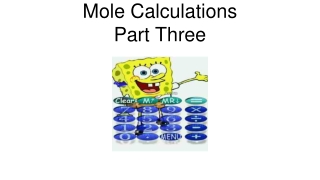DownloadDownload PresentationMole Calculations Part Three

# Mole Calculations Part Three

Télécharger la présentation## Mole Calculations Part Three

- - - - - - - - - - - - - - - - - - - - - - - - - - - E N D - - - - - - - - - - - - - - - - - - - - - - - - - - -
##### Presentation Transcript

1. Mole CalculationsPart Three

2. Calculate the number of O atoms in 250. g of CO2. 250. g CO2 x 1 mole x 6.02 x 1023 molec x 2 atoms O = 6.84 x1024 atoms O 44.0 g 1 mole 1 molec CO2 C O O Note, there are 2 O's for each CO2 molecule

3. There are 10 O atoms in one formula unit O O S2-- Cu2+ O O O O O O O o Octahedron

4. Calculate the mass of CuSO4. 6H2O that contains 6.93 x 1025 atoms of O. x 1 FU x 1 mole 6.93 x 1025 atoms O x 267.72 g = 3.08 x 103 g 10 atoms O 6.02 x 1023 FU 1 mole

5. Calculate the mass of P2Cl5 that contains 2.6 x 1026 atoms of Cl. 2.6 x 1026 atoms Cl x 1 molecs x 1 mole x 239.5 g = 2.1 x104 g 1 mole 5 atoms Cl 6.02 x 1023 molec

6. Calculate the atoms of Cl in 25.0 g ofP2Cl5. 25.0 g P2Cl5 x 1 mole x 6.02 x 1023molec x 5 atoms Cl = 3.14 x 1023 at Cl 1 molec 239.5 g 1 mole